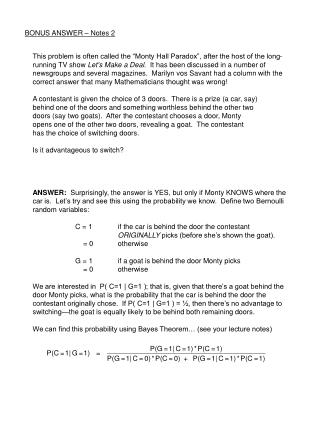Download PresentationBONUS ANSWER – Notes 2

Loading in 2 Seconds...

# BONUS ANSWER – Notes 2 - PowerPoint PPT Presentation

BONUS ANSWER – Notes 2.I am the owner, or an agent authorized to act on behalf of the owner, of the copyrighted work described.
Download Presentation## BONUS ANSWER – Notes 2

An Image/Link below is provided (as is) to download presentation

Download Policy: Content on the Website is provided to you AS IS for your information and personal use and may not be sold / licensed / shared on other websites without getting consent from its author.While downloading, if for some reason you are not able to download a presentation, the publisher may have deleted the file from their server.

- - - - - - - - - - - - - - - - - - - - - - - - - - E N D - - - - - - - - - - - - - - - - - - - - - - - - - -
Presentation Transcript
1. BONUS ANSWER – Notes 2 This problem is often called the “Monty Hall Paradox”, after the host of the long-running TV show Let's Make a Deal. It has been discussed in a number of newsgroups and several magazines. Marilyn vos Savant had a column with the correct answer that many Mathematicians thought was wrong! A contestant is given the choice of 3 doors. There is a prize (a car, say) behind one of the doors and something worthless behind the other two doors (say two goats). After the contestant chooses a door, Monty opens one of the other two doors, revealing a goat. The contestant has the choice of switching doors. Is it advantageous to switch? ANSWER: Surprisingly, the answer is YES, but only if Monty KNOWS where the car is. Let’s try and see this using the probability we know. Define two Bernoulli random variables: C = 1 if the car is behind the door the contestant ORIGINALLY picks (before she’s shown the goat). = 0 otherwise G = 1 if a goat is behind the door Monty picks = 0 otherwise We are interested in P( C=1 | G=1 ); that is, given that there’s a goat behind the door Monty picks, what is the probability that the car is behind the door the contestant originally chose. If P( C=1 | G=1 ) = ½, then there’s no advantage to switching—the goat is equally likely to be behind both remaining doors. We can find this probability using Bayes Theorem… (see your lecture notes)

2. We now just need to figure out all of the probabilities on the right hand side. P(G=1|C=1) = 1 Why? There’s only one car! If the original door chosen by the contestant has a car behind it, then either of the doors Monty chooses will have a goat. P(C=1) = 1/3 When the contestant first picks a door, she is choosing from three doors that are equally likely to have the car behind them—so she has a 1/3 chance of picking the door with the car. P(C=0) = 2/3 Since there are 2 goats and 1 car behind the 3 doors, the probability the contestant picks a door with a goat behind it is 2/3. [Also, note that P(C=0) = 1 – P(C=1).] Now, here’s where the key assumption mentioned in my hints comes into play. If the door the contestant originally picks has a goat behind it (C=0), what is the probability that Monty also picks a door with a goat? The answer is, IT DEPENDS on whether Monty knows where the car is! If he doesn’t, P(G=1|C=0) = ½, because if the door the contestant picks has a goat behind it, Monty is then picking from two doors, one of which has a goat and the other has a car. Basically in this case, we assume that Monty could “accidentally” reveal the car, and the game would end. But if he DOES know where the car is, we assume that Monty will always pick a door with a goat and keep the game going. In this case P(G=1|C=0) = 1. Now if Monty DOESN’T know where the car is, P(G=1|C=0) = ½, and In this case there’s no advantage to switching—there’s a 50% chance of winning either way. But if Monty DOES know where the car is, P(G=1|C=0) = 1 and we have

3. If we assume Monty knows where the car is, then after the contestant is shown the goat, there is only a 1/3 probability that the door she originally chose is the one with the car behind it. That means there is a 2/3 probability the car is behind the other door. So she would definitely want to switch!! There are many other ways to arrive at this solution, and some of them are more intuitive (I wrote this solution because it uses what we’ve done in class). If you’re interested, one good intuitive explanation of the answer is here.